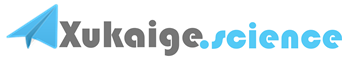double d1 = Math.Round(1.25, 1);//1.2
double d2 = Math.Round(1.24, 1);//1.2
double d3 = Math.Round(1.26, 1);//1.3
double d4 = Math.Round(1.35, 1);//1.4

///<summary>
/// 实现数据的四舍五入法
///</summary>
///<param name="v">要进行处理的数据</param>
///<param name="x">保留的小数位数</param>
///<returns>四舍五入后的结果</returns>
privatedouble Round(double v, int x)
{
bool isNegative =false;
//如果是负数
if (v <0)
{
isNegative =true;
v =-v;
}
int IValue =1;
for (int i =1; i <= x; i++)
{
IValue = IValue *10;
}
double Int = Math.Round(v * IValue +0.5, 0);
v = Int / IValue;
if (isNegative)
{
v =-v;
}
return v;
}

Math.Round ()在四舍五入时有个问题：
Math.Round(2.5,0) = 2;
Math.Round(3.5,0) = 4;
2.5应该等于3才对！

Math.Round()准确的说，这个函数不是四舍五入，而是四舍六入五凑偶，就是说小于4或大于6的该舍该入是没有争议的，而5处在正中间，如果四舍五入则会造成数据的整体偏差，所以采取的原则是：如果舍入位为5，则舍入后最后一位为偶数，这是国际惯例。

C# 中没有四舍五入函数，事实上我知道的程序语言都没有四舍五入函数，因为四舍五入算法不科学，国际通行的是 Banker 舍入法 Banker 's rounding（银行家舍入）算法，即四舍六入五取偶。事实上这也是 IEEE 规定的舍入标准。因此所有符合 IEEE 标准的语言都应该是采用这一算法的
Math.Round 方法默认的也是 Banker 舍入法 在 .NET 2.0 中 Math.Round 方法有几个重载方法
Math.Round(Decimal, MidpointRounding)
Math.Round(Double, MidpointRounding)
Math.Round(Decimal, Int32, MidpointRounding)
Math.Round(Double, Int32, MidpointRounding)

AwayFromZero 当一个数字是其他两个数字的中间值时，会将其舍入为两个值中绝对值较大的值。
ToEven 当一个数字是其他两个数字的中间值时，会将其舍入为最接近的偶数。

Math.Round(3.45, 2, MidpointRounding.AwayFromZero)

double ChinaRound(double valueint decimals)
if (value < 0)
{
return Math.Round(value + 5 / Math.Pow(10, decimals + 1), decimals, MidpointRounding.AwayFromZero);
}
else
{
return Math.Round(value, decimals, MidpointRounding.AwayFromZero);
}

Math.Ceiling（）和Math.Floor
Math.Ceiling(3.1)=4;
Math.Floor(3.9)=3;

floor 和 ceil是math unit 里的函数，使用前要先 Uses Math。
trunc 和 round 是system unit 里的函数，缺省就可以用。
floor 直接往小的取，比如 floor(-123.55)=-124，floor(123.55)=123
trunc 直接切下整数，比如 trunc(-123.55)=-123, floor(123.55)=123
ceil 直接往大的取，比如 ceil(-123.55)=-123， ceil(123.55)=124
round 计算四舍五入，比如 round(-123.55)=-124，round(123.55)=124
C#取整函数向上取整实例
int a = 5;
int b = 2;
lbl.Text = Convert.ToString(Math.Ceiling((double)a / (double)b));